# ILP Part 9 – Conditional operator

This is the ninth part of the ILP series. For your convenience you can find other parts in the table of contents in Part 1 – Boolean algebra

Today we are going to implement conditional operator in ILP. We are basically going to represent if statement in declarative language.

# Interpretation

We will start with explanation what we are going to do. ILP is a declarative paradigm and we are not able to define imperative constructs like loops, conditions, switches etc. We can only define the results for every possible outcome of these operations.
This means that we are not going to define something like ifis greater thanthen set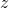to zero. We can’t do that because there is no “flow” in ILP program. Instead of that, we are going to define constructs which will force solver to assign correct values to variables. So we will do something which will guarantee that if in the actual solution it is true that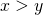thenwill be equal to zero. It is up to the solver whether the condition will be true or false.
You need to remember that we are not able to modify the order of variables binding. You might imagine that solver will first decide whether the condition is true and then will set the value for, however, the solver might as well first decide thatis zero and then make sure that the condition is met.

# Implementation

The actual formula is in fact very easy. Let’s define variables first: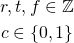We have condition named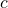which can be only true or false. We also have values for true () value and false (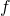) value.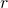represents the result of the calculation.
We want to achieve the following: if in final solutionis equal tothenshould be equal to. Otherwise,should be equal to. In C++ you would represent this as:
r = c ? t : f;
The formula is pretty easy: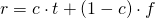Whenis one we get: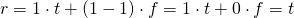. However, whenis false we get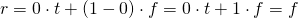.
We already know how to multiply variables so we are able to calculate this formula. In fact it is even easier because we can use the trick with minimum value instead of multiplication, however, this is only a matter of efficiency.

# Summary

Today we have implemented our first imperative construct in ILP. In one of the next parts we will see how to use it to implement loops. Stay tuned!